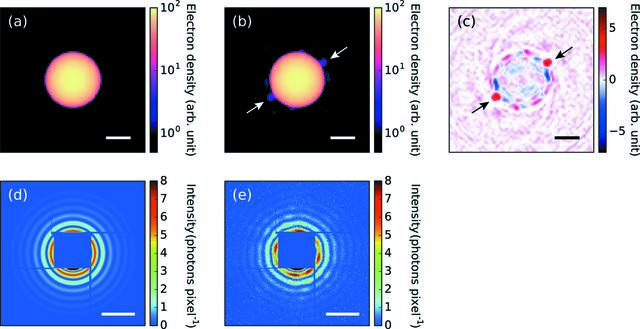disable zoom     view article Figure 6 Result of the structure reconstruction from the measured diffraction pattern [Fig. 1(c)] using the implemented algorithm. (a) A projection of a uniform dense sphere used as the initial estimate of the density map. (b) The reconstructed density map by the implemented algorithm. (c) The difference between (a) and (b) defined as (b) − (a). (d) The simulated pattern from (a). (e) The simulated pattern from (b). The scale bars in (a)–(c) are 100 nm and those in (d)–(e) are 0.1 nm−1.

IUCrJ
ISSN: 2052-2525